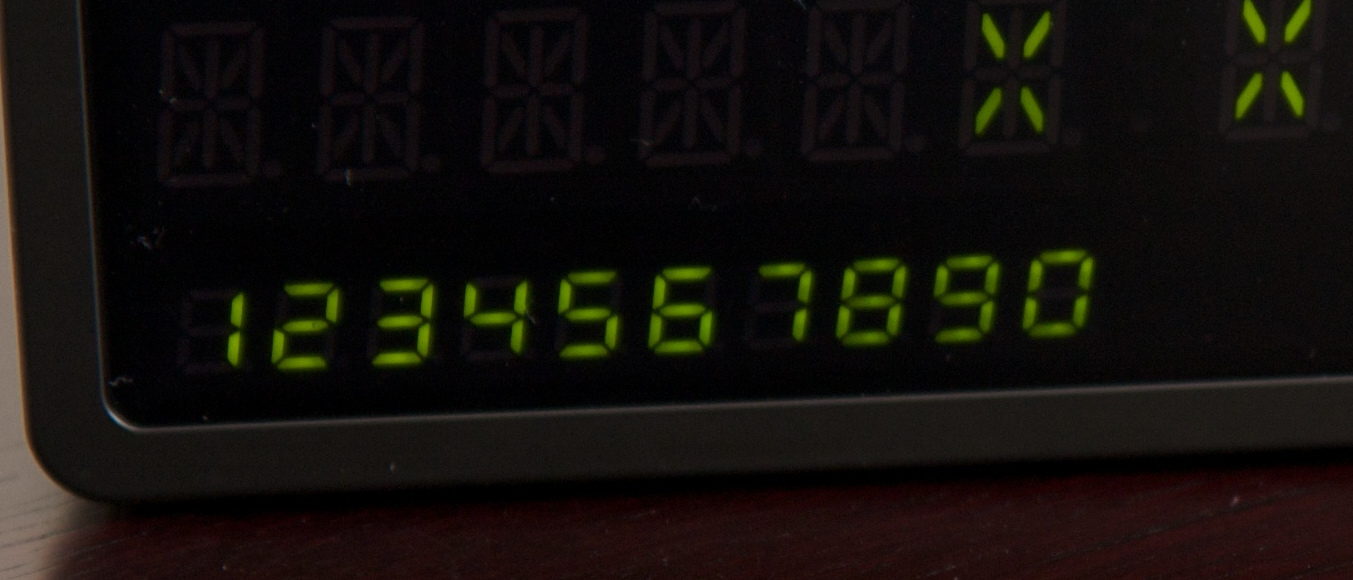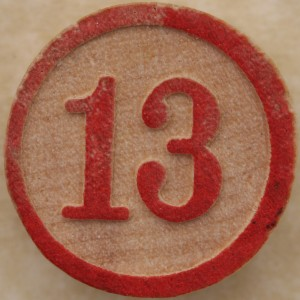# Pandigital square numbers

Why are there none in base 13?A square number containing every digit from 0 to 9 exactly once.
Chalkdust Prize Crossnumber #1, 5 down

Whilst trying to answer 5 down in the Chalkdust crossnumber, I discovered that there are 87 square numbers in base 10 that use every digit exactly once (without a leading zero). This made me wonder how many square numbers have this property in other number bases.

I quickly wrote some code to get an idea of how many pandigital (without repeating digits or a leading zero) squares there are in other number bases.

### What is a base?Numbers in binary, or base 2.

When we write numbers, we usually use the rightmost digit to represent 1s, the next 10s, the next 100s and so on. This way of writing number is called base ten, as each digit represents the next power of 10. We could write numbers in another base: for example, if we write numbers in base 4, then digits (starting from the right) represent 1s, 4s, 16s, 64s and so on. The number 1302 in base 4 is equal to $2+0\times 4+3\times 4^2+1\times 4^3$, or 114 (in base 10).

Throughout this article, I will use subscripts to represent bases. For example, $12_3$ is 12 in base 3 (5 in base 10).

### Through the Bases

In bases 2 and 3, I found that there are 0 pandigital squares. This isn’t surprising as there’s only one pandigital binary number, $10_2$ (better known as the number 2), and it’s not square. In base 3, there are only a few pandigital numbers: $102_3$, $120_3$, $201_3$ and $210_3$, so it’s not too much of a mystery that we don’t hit a square.

In base 4, I found my first pandigital square: $3201_4$, which in base 10 is $225=15 \times 15$. This is the only pandigital square in base 4. The number of pandigital squares continues like so:
Base 5: 0
Base 6: 1
Base 7: 3
Base 8: 4
Base 9: 26
Base 10: 87
Base 11: 47
Base 12: 87

Great! I was starting to find some pandigital squares.

Then base 13 came along. The code found no pandigital squares in base 13. The results had been ticking up slowly as the code hunted the square numbers. Zero results for base 13 seemed plain weird.

The obvious explanation is usually a bug in the code, but the sun was shining and I was sick of sitting behind a keyboard. Instead of reading code, I took a pencil and notepad outside and began to look at pandigital numbers…

### Why there are no base 13 pandigital squaresLet $p$ be a pandigital number with no repeating digits and without a leading zero in base $n$. Let $a_i$ be its digits. $p$ can be written as $$a_{n-1}n^{n-1} + a_{n-2}n^{n-2}+…+a_1n^1+a_0n^0$$ or
$$\sum_{i=0}^{n-1}{a_in^i}.$$
This can be rewritten as
$$p=\sum_{i=0}^{n-1}{a_i} + \sum_{i=0}^{n-1}{a_i(n^i-1)}.$$

Let $R_i^{(n)}$ represent the $i$-digit repunit (repeating unit) number $11…11_n$. $R_i^{(n)}$ can be written as $\dfrac{n^i-1}{n-1}$. This means that $$p=\sum_{i=0}^{n-1}{a_i} + \sum_{i=0}^{n-1}{a_i(n-1)R_i^{(n)}}.$$

$\sum\limits_{i=0}^{n-1}{a_i}$ is the sum of $p$’s digits and (for pandigitals) a simple arithmetic series, for which we have an easy expression:
$$p=\frac{n(n-1)}{2} + \sum_{i=0}^{n-1}{a_i(n-1)R_i^{(n)}}$$
$$=\frac{n(n-1)}{2} + (n-1)\sum_{i=0}^{n-1}{a_iR_i^{(n)}}$$

When $n$ is odd it makes sense to extract the factor $\dfrac{n-1}{2}$, as this is an integer.
$$p=\frac{n-1}{2}\left(n+2\sum_{i=0}^{n-1}{a_iR_i^{(n)}}\right)$$

Since $n$ is odd and $2\sum\limits_{i=0}^{n-1}{a_iR_i^{(n)}}$ is even, $\left(n+2\sum\limits_{i=0}^{n-1}{a_iR_i^{(n)}}\right)$ must be odd.

If $\dfrac{n-1}{2}$ contains an odd number of $2$s as prime factors, and knowing that the odd number $\left(n+2\sum\limits_{i=0}^{n-1}{a_iR_i^{(n)}}\right)$ can’t contribute any $2$s, we are left with an odd number of $2$s as prime factors of $p$. But an odd number of 2s cannot be split equally in half to make a square root. So $p$ cannot be a square.

Getting back to base 13. When $n=13$ then $\dfrac{13-1}{2}=6=2 \times 3$, and because there’s only one 2, there are no pandigital squares in base 13.

For the same reason, there will be no pandigital square numbers in bases 17, 49, 81 or many other bases.

Chalkdust’s crossnumber question wasn’t about base 13, but it does show the fun of recreational mathematics; every answer suggests another question.

If you would like to learn more about the number of pandigital square numbers in base $n$, or extend the sequence, then you can find the sequence on the Online Encyclopedia of Integer Sequences. If you have not yet entered the Crossnumber competition, there is still time.Adam is a middle aged software developer who enjoys recreational maths. He spends so much reading hex and binary that he doesn’t take base $10_{10_{…}}$ as given anymore.
@ajtpartridge    apartridge.com/    + More articles by Adam